# GRE Subject Test: Chemistry : Metals

## Example Questions

### Example Question #2 : Periodic Table Groupings

An unknown element has been studied in the lab. It has been shown to be malleable, ductile, and a good conductor of heat. Which element best fits this description?

Cobalt

Boron

Sulfur

Silicon

Cobalt

Explanation:

The correct answer is cobalt, since it is the only metal among the answer choices. Metals have all the properties described (malleability, ductility, and conductivity). Sulfur, boron, and silicon do not exhibit these properties to the same extent.

### Example Question #1 : Elements And The Periodic Table

There is a unknown element in a jar. It is a solid at room temperature and conducts electricity. Which of the following could be true about this element?

The identity of the element is silicon and it is a good oxidizing agent

The identity of the element is silicon and it can be stretched to create a thin wire

The identity of the element is sodium and it is a good oxidizing agent

The identity of the element is sodium and it can be stretched to create a thin wire

The identity of the element is sodium and it can be stretched to create a thin wire

Explanation:

The question states that the element is a solid at room temperature and conducts electricity. These are characteristics of a metal; therefore, the element is likely a metal. Recall that metals are usually found on the left side of the periodic table. The groups that are classified as metals include the alkali metals (group 1), alkaline earth metals (group 2), the aluminum family (group 3), and the transition metals (D block).

The answer choices state that it could be either sodium or silicon. Silicon is in group 14 and is considered a non-metal; therefore, the element has to be sodium. Metals are good electrical conductors and solid at room temperature. They are also ductile, which means that a metal can be stretched to create thin wires.

Metals are good reducing agents, not oxidizing agents. Recall that metals usually have one, two, or three valence electrons. Since they only have a few valence electrons, metals prefer to lose their valence electrons to complete an octet. When an element loses electrons it is considered to be oxidized and can act as a reducing agent; therefore, sodium is not an oxidizing agent.

### Example Question #52 : Atoms, Elements, And The Periodic Table

What class of element is zinc?

Alkali metal

Transition metal

Metalloid

Non-metal

Transition metal

Explanation:

In general, the metals fall on the left side of the periodic table and are separated from the non-metals by the metalloids. Transition metals fall in the d block of the periodic table, in groups (columns) 3-12. Examples of metals, non-metals, transition metals, and metalloids are calcium, oxygen, zinc, and arsenic, respectively.

Alkali metals are a special class of metal only found in group 1 of the periodic table.

### Example Question #3 : Gre Subject Test: Chemistry

The multiple oxidation states of transition metals are due to which of the following properties?

The large number of valence electrons

The presence of incomplete d orbitals

The instability of the p orbitals

The weak attraction between the nucleus and its core electrons

The presence of incomplete d orbitals

Explanation:

The transition metals are defined in the region of the periodic table in which atoms are being added to the d subshell. As a result, the transition metals have unfilled or incomplete orbitals within the d shell. Since each orbital is filled with one electron before orbitals start to become completely filled, there are increasing numbers of unpaired d shell orbitals. This allows transition metals to give up variable numbers of electrons, while maintaining stability, as electrons move between d orbitals.

A common example is iron, which is stable in both the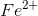and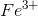electron configurations.

### Example Question #4 : Gre Subject Test: Chemistry

Which of the following is true about transition metals?

I. Transition metals form acidic oxides

II. A transition metal can have multiple oxidation states

III. Transition metals can be reduced, but can never be oxidized

II only

I only

I and II

I, II, and III

II only

Explanation:

Transition metals are classified as metals; therefore, the oxides they form are called metal oxides. Metal oxides are basic compounds, whereas non-metal oxides are acidic compounds. Metal oxides, such as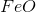and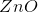, give rise to high pH values, and non-metal oxides, such as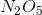and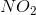, give rise to low pH values.

An oxidation state is defined as the degree of oxidation a compound can achieve. It is often calculated by observing the gain and loss of electrons in an atom. For example, an atom that loses two electrons will have an oxidation number of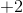, whereas an atom that gains two electrons will have an oxidation number of. Some transition metals have multiple oxidation states because they can lose varying amounts of electrons. This occurs because the energy difference between the outermostorbital and the outermostorbital is small; therefore, the energy required to remove the electron from the subsequentorbital is comparable to removing electrons from theorbital.

For example, iron (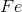) can have an oxidation state ofor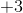. Iron’s electron configuration is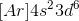. When it loses two electrons, iron will have an empty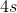orbital. The electron configuration ofwill be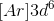. The amount of energy difference between the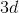orbital and theorbital is very small; therefore, it is easy for iron to lose another electron from theorbital and become. On the other hand, it is very hard to remove electrons from a filledorbital. This explains why non-metals, such as the oxygen and halogen groups, and metals, such as alkali and alkaline earth metals, have only one oxidation state.

Reduction involves gaining electrons and oxidation involves losing electrons. This means that reduction will decrease the oxidation number and oxidation will increase the oxidation number. It is generally favorable for a transition metal to lose electrons and become oxidized, though reduction can be achieved by adding enough energy to the system (such as in a electrolytic cell).

### All GRE Subject Test: Chemistry Resources